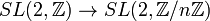# Special linear group:SL(2,Z)

Jump to: navigation, search
This article is about a particular group, i.e., a group unique upto isomorphism. View specific information (such as linear representation theory, subgroup structure) about this group
View a complete list of particular groups (this is a very huge list!)[SHOW MORE]

## Definition

The group$SL(2,\mathbb{Z})$ is defined as the group, under matrix multiplication, of$2 \times 2$ matrices over$\mathbb{Z}$, the ring of integers, having determinant$1$.

In other words, it is the group with underlying set:$\left \{ \begin{pmatrix} a & b \\ c & d \\\end{pmatrix} \mid a,b,c,d \in \mathbb{Z}, ad - bc = 1 \right \}$

This is the degree two case of a special linear group over integers and hence of a special linear group. It is also a special case of a special linear group of degree two.

The group also has the following equivalent descriptions:

### Definition by presentation

The group can be defined by any of the following presentations (here,$1$ denotes the identity element):

• PLACEHOLDER FOR INFORMATION TO BE FILLED IN: [SHOW MORE]
• From the amalgamated free product definition:$\langle x,y \mid x^4 = 1, x^2 = y^3 \rangle$

### Structures

Thinking of$SL(2,\mathbb{Z})$ as a group of matrices, we see that it is an example of an arithmetic group.

## Arithmetic functions

Function Value Explanation
order infinite (countable) As$SL(2,\mathbb{Z})$: The group is infinite because, for instance, it contains all matrices of the form$\begin{pmatrix} 1 & a \\ 0 & 1 \\\end{pmatrix}$ for$a \in \mathbb{Z}$.
As a set, the group is contained in the set of all$2 \times 2$ matrices over$\mathbb{Z}$. This can be identified with$\mathbb{Z}^4$, which is countable since$\mathbb{Z}$ is countable. Thus,$SL(2,\mathbb{Z})$ is also countable.
As an amalgamated free product: any amalgamated free product relative to a subgroup that is proper in both groups is infinite.
exponent infinite As$SL(2,\mathbb{Z})$: The group contains the element$\begin{pmatrix} 1 & 1 \\ 0 & 1 \\\end{pmatrix}$, which has infinite order.
minimum size of generating set 2 As$SL(2,\mathbb{Z})$: Follows from elementary matrices of the first kind generate the special linear group over a Euclidean ring, so$SL(2,\mathbb{Z})$ is generated by all matrices of the form$\begin{pmatrix} 1 & a \\ 0 & 1 \\\end{pmatrix}$ and$\begin{pmatrix} 1 & 0 \\ b & 1 \\\end{pmatrix}$ with$a,b$ varying over$\mathbb{Z}$. By the fact that the additive group of$\mathbb{Z}$ is cyclic, all these matrices are generated by$\begin{pmatrix} 1 & 1 \\ 0 & 1 \\\end{pmatrix}$ and$\begin{pmatrix} 1 & 0 \\ 1 & 1 \\\end{pmatrix}$.
As an amalgamated free product of two cyclic groups: follows that it is 2, just from the definition (note that the generators here are different from those used in the justification in matrix terms).
subgroup rank infinite (countable)$SL(2,\mathbb{Z})$ has a subgroup that is isomorphic to free group:F2 (see Sanov subgroup in SL(2,Z) is free of rank two). This in turn has free subgroups of countable rank.

## Group properties

Property Satisfied? Explanation Corollary properties satisfied/dissatisfied
2-generated group Yes See explanation for minimum size of generating set above satisfies: finitely generated group, countable group
Noetherian group No See explanation for subgroup rank above
finitely presented group Yes Any of the definitions ($SL$, B_amalgamated free product) gives a finite presentation
solvable group No contains subgroup isomorphic to free group:F2 -- see Sanov subgroup in SL(2,Z) is free of rank two dissatisfies: nilpotent group, abelian group
group satisfying no nontrivial identity Yes contains subgroup isomorphic to free group:F2 -- see Sanov subgroup in SL(2,Z) is free of rank two
SQ-universal group Yes contains subgroup isomorphic to free group:F2 -- see Sanov subgroup in SL(2,Z) is free of rank two
residually finite group Yes The kernels of the homomorphisms$SL(2,\mathbb{Z}) \to SL(2,\mathbb{Z}/n\mathbb{Z})$ for natural numbers$n$ are normal subgroups of finite index and their intersection is trivial. satisfies: finitely generated residually finite group
Hopfian group Yes Follows from finitely generated and residually finite implies Hopfian satisfies: finitely generated Hopfian group

## Elements

Further information: element structure of special linear group:SL(2,Z)

## Facts

• Sanov subgroup in SL(2,Z) is free of rank two
• The homomorphism$SL(2,\mathbb{Z}) \to SL(2,\mathbb{Z}/n\mathbb{Z})$ is surjective for any natural number$n$.

## GAP implementation

Description Functions used
SL(2,Integers) SL, Integers